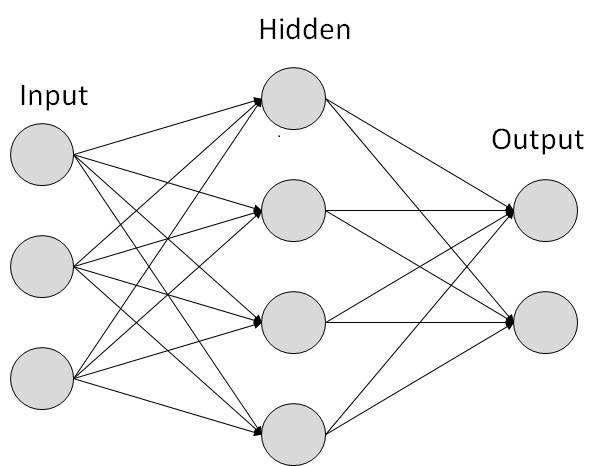# Which layer do synapses belong to? (before or after)

Say I have an ANN with 1 input, 1 hidden and 1 output layer. When I am referring to 'parameters' or 'weights' in the hidden layer, are they between input - hidden or hidden - output? I also saw some conflicting info on what a layer is: In correct science is this just neurons or just synapses or some combination between the two? Thank you.

If I understood your question correctly, you want to know which layer do the weights and biases (or lines that connect the nodes in the following figure) belong to:The answer is they belong to the layer they are going to, not the one from which they are coming from.

Explanation:
In a neural network:

• The input layer has no parameters (it just contains the network's input). We'll refer to this layer as $X$.
• The hidden layer has a weight matrix (we'll call it $W_h$ whose dimensions are $(number\_of\_ inputs \;\cdot \; number\_of\_neurons)$, a bias matrix ($b_h$) whose dimensions are $(number\_of\_neurons)$ and an activation function ($f_h$). The output of this layer is $y_h = f_h(W_h \cdot X + b_h)$. The weights and biases between the input and hidden layer clearly belong to this layer.
• The output layer has a weight matrix ($W_o$) whose dimensions are $(number\_of\_ neurons \;\cdot \; number\_of\_outputs)$, a bias matrix ($b_o$) whose dimensions are $(number\_of\_outputs)$ and an activation function ($f_o$). The output of this layer is $\hat y = f_o(W_0 \cdot y_h + b_o)$. The weights and biases connecting the hidden with the output layer belong to this layer.
• I would add that the picture is really just a diagram of a computation. The lines indicate where the inputs come from, and each circle is a function that creates a single output. The weights, bias, and activation are what happens inside the circle. It's not a very detailed schematic. – Aleksandr Dubinsky Apr 22 '18 at 0:28

When I am referring to 'parameters' or 'weights' in the hidden layer, are they between input - hidden or hidden - output?

Between both.

I also saw some conflicting info on what a layer is: In correct science is this just neurons or just synapses or some combination between the two?

I have no idea what you call "correct science", but ANNs do not model real neuronal networks, it's a metaphor, a meta-heuristic. Having said that, the idea is that the nodes in the layers are neurons themselves. They receive input from previous layers and pass it forward, so a layer is just like a cortical layer so common in the cortex: it's a collection of neurons organized, just in a more contrived way in an ANNs than in real biology.